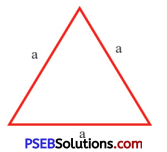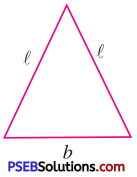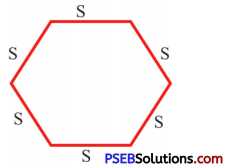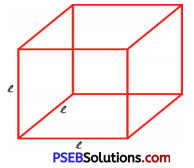# PSEB 6th Class Maths Solutions Chapter 7 Algebra Ex 7.2

Punjab State Board PSEB 6th Class Maths Book Solutions Chapter 7 Algebra Ex 7.2 Textbook Exercise Questions and Answers.

## PSEB Solutions for Class 6 Maths Chapter 7 Algebra Ex 7.2

1. Each side of equilateral triangle is denoted by ‘a’ then express the perimeter of the triangle using ‘a’.Solution:
Each triangle of equilateral triangle = a
∴ Perimeter of equilateral triangle
= a + a + a = 3a2. An isosceles triangle is shown. Express its perimeter in terms of ‘l’ and ‘b’.Solution:
Perimeter of isosceles triangle = l + l + b
= 21 + b

3. Each side of regular hexagon is denoted by ‘S’ then express the perimeter of the regular hexagon using ‘S’.Solution:
Each side of regular hexagon = S
Perimeter of regular hexagon
=S + S + S + S + S + S
= 6S4. The cube has 6 faces and all of them are identify squares. If l is the length of an edge of a cube, find the total length of all edges of the cube in terms of ‘l’?Solution:
Length of each edge of a cube = l
There are 12 edges of a cube
Total length of all edges of the cube
= 12 × l = 12l

5. Write commutative property of addition using variables x and y.
image
Solution:
According to commutative property of addition.
If the order of numbers, in addition, is changed it does not change their sum.
∴ x + y = y + x6. Write associative property of multiplication using variables l, m and n.
Solution:
According to associative property of multiplication.
If three numbers can be multiplied in any order, it does not change their product.
∴ l × (m × n) = (l × m) × n

7. Write distributive property of multiplication over addition in terms of variables p, q and r respectively.
Solution:
According to Distributive property of multiplication over addition
p × (q + r) = p × q + p × r